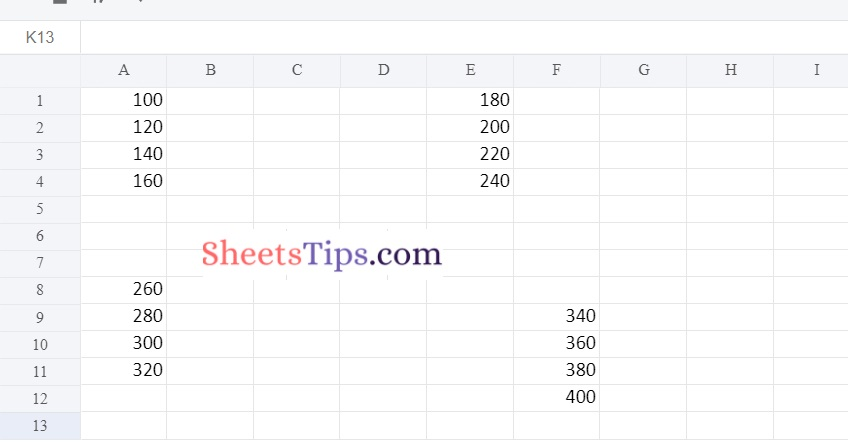# Working with Pandas and XlsxWriter in Python | Set – 1

Pandas is a data analysis library written in Python. It can read, filter, and re-arrange small and large datasets in a variety of formats, including Excel.

Pandas use the XlsxWriter modules to write Excel files.

Also Read: Python Programming Examples with Output

XlsxWriter Module in Python

XlsxWriter is a Python module that allows you to write files in the XLSX file format. It can be used to fill several spreadsheets with text, numbers, and formulas. It also allows formatting, images, charts, page setup, auto filters, conditional formatting, and many more features.

Installation:

Use the following command to install the XlsxWriter module.

`pip install xlsxwriter`
Output:
```Looking in indexes: https://pypi.org/simple, https://us-python.pkg.dev/colab-wheels
/public/simple/
Collecting xlsxwriter
|████████████████████████████████| 149 kB 5.3 MB/s
Installing collected packages: xlsxwriter
Successfully installed xlsxwriter-3.0.3```

#### DataFrame.to_excel() in Pandas:

Using the to_excel() function, we can export the DataFrame to an Excel file.

The target file name must be specified when writing a single object to an excel file. If we wish to write to many/multiple sheets, we must first create an ExcelWriter object with the target filename and then indicate the sheet in the file we want to write to.

The unique sheet_name can also be used to write multiple sheets. All modifications made to the data written to the file must be saved.

NOTE:

```If we create an ExcelWriter object with a file name that already exists,
it will erase and overwrite the existing file's content.
```

Syntax:

```DataFrame.to_excel(excel_writer, sheet_name='Sheet1', na_rep='', float_format=None,
columns=None, header=True, index=True, index_label=None, startrow=0, startcol=0,
engine=None, merge_cells=True, encoding=None, inf_rep='inf', verbose=True,
freeze_panes=None)```

Parameters

• excel_writer: It is a path of an Excel file or an existing ExcelWriter.
• sheet_name: It is the name of the sheet containing the DataFrame.
• na_rep: It is the Missing Data representation.
• float_format: This is optional. It formats the string for floating-point numbers. (ex: float_format= “%.2f”, formats 0.1316 to 0.13)
• columns: These are the columns to write.
• header: The header argument writes out the column names. Suppose, If a list of the string is given, it is assumed to be the aliases for the column names.
• index: This is Boolean. If it is set to True, it writes the index else NOT.
• index_label: It indicates the column label for the index column. If it is not given and both the header and the index are True, then the index names are used. If DataFrame uses MultiIndex, a sequence must be specified.
• startrow: The default value is 0. The upper left cell row to dump the DataFrame.
• startcol: The default value is 0.  The upper left cell column to dump the DataFrame.
• engine: This is optional. It Writes the engine to use, ‘openpyxl’ or ‘xlsxwriter’. This can also be set using the options io.excel.xlsx.writer, io.excel.xls.writer, and io.excel.xlsm.writer.
• merge_cells: This is a Boolean value. The default value is True. It is used to write MultiIndex and Hierarchical rows as the merged cells.
• encoding: This is optional. It encodes the output excel file. It is just required for the xlwt.
• inf_rep: This is optional. The default value is `inf`. In most cases, it represents infinity.
• verbose: This is a Boolean value. The default value is True. It Displays more information in the error logs.
• freeze_panes: This is optional. It represents the one-based bottommost row and rightmost column that is to be frozen.

## Working with Pandas and XlsxWriter in Python

### Method #1: Using Pandas and XlsxWriter to Convert a Pandas dataframe to an xlsx file

Approach:

• Import pandas module using the import keyword
• Create a dataframe by passing some random data to the DataFrame() function of the pandas module and store it in a variable
• Pass the excel file path, engine as xlsxwriter as arguments to the ExcelWriter() function of the pandas module to create an excel writer object.
• Apply to_excel() function on the above dataframe by passing the above writer object, sheet name as arguments to it to write the above dataframe into the worksheet.
• Save the above writer object using the save() function.
• The Exit of the Program.

Below is the implementation:

```# Import pandas module using the import keyword
import pandas as pd

# Create a dataframe by passing some random data to the DataFrame() function
# of the pandas module and store it in a variable
datafrme = pd.DataFrame({'Data': ['Hello', 'this', 'is', 'SheetsTips',
'Welcome', 'all']})

# Pass the excel filepath, engine as xlsxwriter as arguments to the
# ExcelWriter() function of the pandas module to create a excel writer object.
writerObj = pd.ExcelWriter('sampleExcelFile.xlsx',
engine ='xlsxwriter')

# Apply to_excel() function on the above dataframe by passing the above writer object,
# sheet name as arguments to it to Write the above dataframe into the worksheet.
datafrme.to_excel(writerObj, sheet_name ='Sheet1')

# save the above writer object using the save() function
writerObj.save()
```

Output: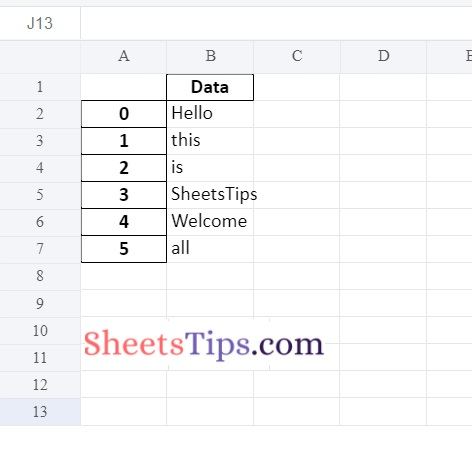### Method #2: Using Pandas and XlsxWriter Modules to Write multiple dataframes to worksheets

Approach:

• Import openpyxl module using the import keyword
• Create a first dataframe by passing some random data to the DataFrame() function
of the pandas module and store it in a variable
• Create a second dataframe by passing some random data to the DataFrame() function of the pandas module and store it in another variable
• Similarly, create 3rd dataframe and store it in another variable
• Pass the excel filepath, engine as xlsxwriter as arguments to the ExcelWriter() function of the pandas module to create an excel writer object.
• Store it in another variable
• Apply to_excel() function on the above first dataframe by passing the above writer object,
and sheet name as arguments to it to write the above first dataframe into the first worksheet.
• Similarly, write each dataframe into different worksheets.
• Save the above writer object using the save() function.
• The Exit of the Program.

Below is the implementation:

```# Import openpyxl module using the import keyword
import openpyxl

# Create a first dataframe by passing some random data to the DataFrame() function
# of the pandas module and store it in a variable
datafrme_1 = pd.DataFrame({'Data': [100, 120, 140, 160]})
# Create a second dataframe by passing some random data to the DataFrame() function
# of the pandas module and store it in another variable
datafrme_2 = pd.DataFrame({'Data': [180, 200, 220, 240]})
# Similarly create 3rd dataframe and store it in another variable
datafrme_3 = pd.DataFrame({'Data': [260, 280, 300, 320]})

# Pass the excel filepath, engine as xlsxwriter as arguments to the
# ExcelWriter() function of the pandas module to create an excel writer object.
# Store it in another variable
engine ='xlsxwriter')

# Apply to_excel() function on the above first dataframe by passing the above writer object,
# sheet name as arguments to it to Write the above first dataframe into the first worksheet.
datafrme_1.to_excel(writerObj, sheet_name ='Sheet1')
# Similarly write each dataframe into different worksheets.
datafrme_2.to_excel(writerObj, sheet_name ='Sheet2')
datafrme_3.to_excel(writerObj, sheet_name ='Sheet3')

# Save the above writer object using the save() function
writerObj.save()
```

Output:

Sheet1: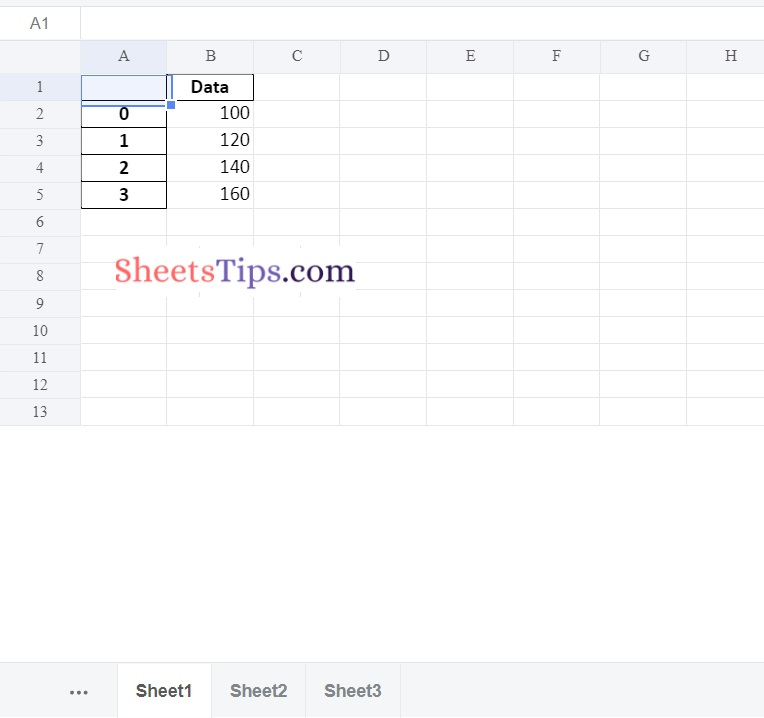Sheet2: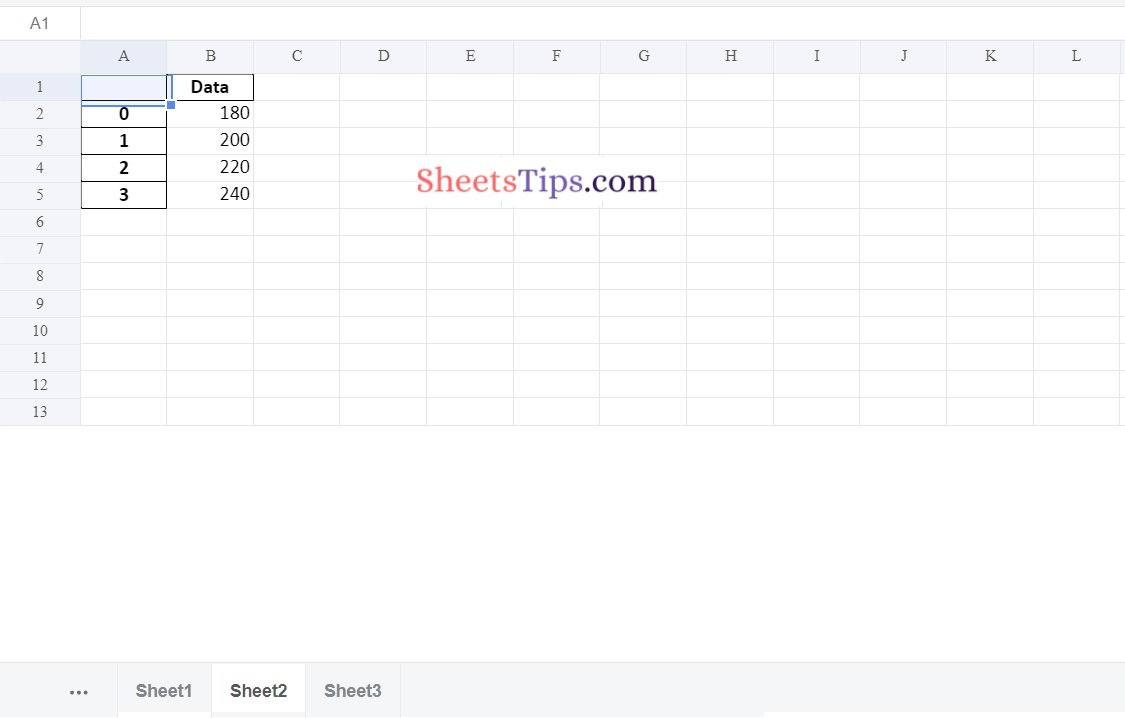Sheet3:### Method #3: Using Pandas and XlsxWriter Modules for Positioning dataframes in a worksheet

Approach:

• Import pandas module using the import keyword
• Import openpyxl module using the import keyword
• Create a first data frame by passing some random data to the DataFrame() function of the pandas module and store it in a variable
• Create a second data frame by passing some random data to the DataFrame() function of the pandas module and store it in another variable
• Similarly, create 3rd and 4th data frames and store them in separate variables
• Pass the excel filepath, engine as xlsxwriter as arguments to the ExcelWriter() function of the pandas module to create an excel writer object.
• Store it in another variable
• Apply to_excel() function on the above first data frame by passing the above writer object, sheet name as arguments to it to write the above first data frame into the first worksheet.
• The default position is A1 cell
• Place the second data frame in the sheet1 at the 5th column(0 indexing) using the startcol attribute.
• Similarly, Place the third data frame in sheet1 at the 8th row using the startrow attribute.
• Add the 4th data frame without header and index by giving header,index arguments as False Values
• Save the above Excel writer object using the save() function.
• The Exit of the Program.

Below is the implementation:

```# Import pandas as pd
import pandas as pd
# Import openpyxl module using the import keyword
import openpyxl
# Create a first dataframe by passing some random data to the DataFrame() function
# of the pandas module and store it in a variable
datafrme_1 = pd.DataFrame({'Data': [100, 120, 140, 160]})
# Create a second dataframe by passing some random data to the DataFrame() function
# of the pandas module and store it in another variable
datafrme_2 = pd.DataFrame({'Data': [180, 200, 220, 240]})
# Similarly create 3rd and 4th dataframes and store them in separate variables
datafrme_3 = pd.DataFrame({'Data': [260, 280, 300, 320]})
datafrme_4 = pd.DataFrame({'Data': [340, 360, 380, 400]})

# Pass the excel filepath, engine as xlsxwriter as arguments to the
# ExcelWriter() function of the pandas module to create an excel writer object.
# Store it in another variable
writerObj  = pd.ExcelWriter('PositioningDataframes.xlsx',
engine ='xlsxwriter')

# Apply to_excel() function on the above first dataframe by passing the above writer object,
# sheet name as arguments to it to write the above first dataframe into the first worksheet.
# The default position is A1 cell
datafrme_1.to_excel(writerObj , sheet_name ='Sheet1')
# Place the second dataframe in the sheet1 at the 5th column(0 indexing) using the
# startcol attribute
datafrme_2.to_excel(writerObj , sheet_name ='Sheet1', startcol = 4)
# Similarly, Place the third dataframe in the sheet1 at the 8th row using the
# startrow attribute
datafrme_3.to_excel(writerObj , sheet_name ='Sheet1', startrow = 7)

datafrme_4.to_excel(writerObj , sheet_name ='Sheet1',
startrow = 8, startcol = 5,
header = False, index = False)

# Save the above Excel writer object using the save() function
writerObj.save()```

Output: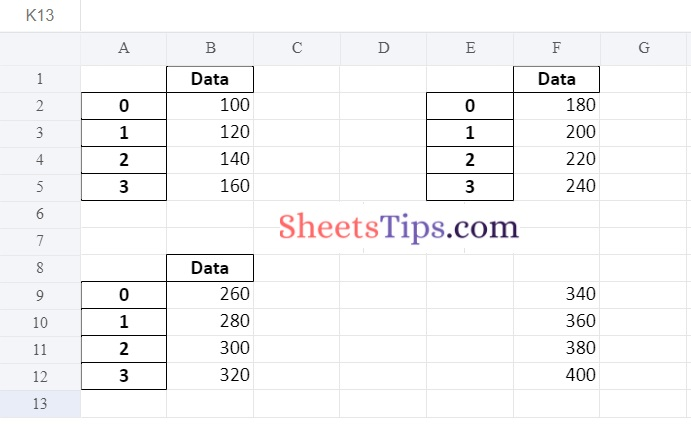### Method #4: Using index & Header as False Argument to remove Indexes and Headers of all Positioning DataFrames

Here by using the index= False argument of the to_excel() function, we can remove the indexes in an excel file.

Approach:

• Import pandas module using the import keyword
• Import openpyxl module using the import keyword
• Create a first data frame by passing some random data to the DataFrame() function of the pandas module and store it in a variable
• Create a second data frame by passing some random data to the DataFrame() function of the pandas module and store it in another variable
• Similarly, create 3rd and 4th data frames and store them in separate variables
• Pass the excel filepath, engine as xlsxwriter as arguments to the ExcelWriter() function of the pandas module to create an excel writer object.
• Store it in another variable
• Apply to_excel() function on the above first data frame by passing the above writer object, and sheet name as arguments to it and also to add without header and index by giving header, index arguments as False Values.
• The default position is A1 cell
• Place the second data frame in the sheet1 at the 5th column(0 indexing) using the startcol attribute.
• Similarly, Place the third data frame in sheet1 at the 8th row using the startrow attribute.
• Add the 4th data frame without header and index by giving header,index arguments as False Values
• Save the above Excel writer object using the save() function.
• The Exit of the Program.

Below is the implementation:

```# Import pandas as pd
import pandas as pd
# Import openpyxl module using the import keyword
import openpyxl
# Create a first dataframe by passing some random data to the DataFrame() function
# of the pandas module and store it in a variable
datafrme_1 = pd.DataFrame({'Data': [100, 120, 140, 160]})
# Create a second dataframe by passing some random data to the DataFrame() function
# of the pandas module and store it in another variable
datafrme_2 = pd.DataFrame({'Data': [180, 200, 220, 240]})
# Similarly create 3rd and 4th dataframes and store them in separate variables
datafrme_3 = pd.DataFrame({'Data': [260, 280, 300, 320]})
datafrme_4 = pd.DataFrame({'Data': [340, 360, 380, 400]})

# Pass the excel filepath, engine as xlsxwriter as arguments to the
# ExcelWriter() function of the pandas module to create an excel writer object.
# Store it in another variable
engine ='xlsxwriter')

# Apply to_excel() function on the above first dataframe by passing the above writer object,
# sheet name as arguments to it to write the above first dataframe into the first worksheet.
# The default position is A1 cell
datafrme_1.to_excel(writerObj , sheet_name ='Sheet1',header = False, index = False)
# Place the second dataframe in the sheet1 at the 5th column(0 indexing) using the
# startcol attribute
datafrme_2.to_excel(writerObj , sheet_name ='Sheet1', startcol = 4,header = False, index = False)
# Similarly, Place the third dataframe in the sheet1 at the 8th row using the
# startrow attribute
datafrme_3.to_excel(writerObj , sheet_name ='Sheet1', startrow = 7,header = False, index = False)